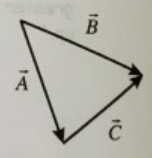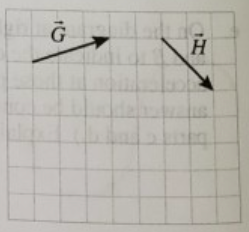# Problem: Part A.Write a vector equation (e.g., D + E + F = 0) that relates the vectors A, B, and C shown at right.Part B.On the grid at right, draw a diagram that shows how to find the vector I that must be added to G to give the vector H. Clearly label this vector.

###### FREE Expert Solution

Part A

We need to apply triangle law in this part.

According to the triangle law, two vectors can be represented by two sides of a triangle in the same order.

This is where the head of a vector joined at the tail of another.

90% (286 ratings)###### Problem Details

Part A.

Write a vector equation (e.g., D + E + F = 0) that relates the vectors A, B, and C shown at right.Part B.

On the grid at right, draw a diagram that shows how to find the vector I that must be added to G to give the vector H. Clearly label this vector.